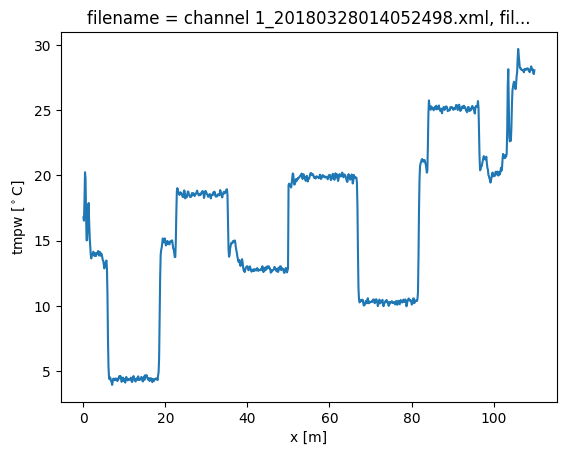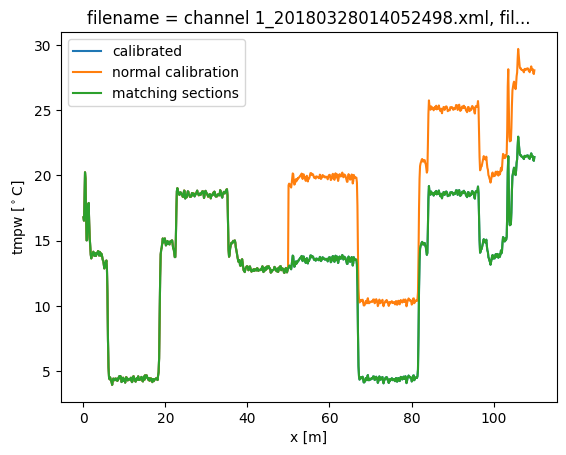# 15. Calibration using matching sections¶

In notebook 14 we showed how you can take splices or connectors within your calibration into account. To then calibrate the cable we used reference sections on both sides of the splice. If these are not available, or in other cases where you have a lack of reference sections, matching sections can be used to improve the calibration.

For matching sections you need two sections of fiber than you know will be the exact same temperature. This can be, for example, in duplex cables or twisted pairs of cable.

## Demonstration¶

To demonstrate matching sections, we’ll load the same dataset that was used in previous notebooks, and modify the data to simulate a lossy splice, just as in notebook 14.

:

import os

import matplotlib.pyplot as plt

%matplotlib inline

:

filepath = os.path.join("..", "..", "tests", "data", "double_ended2")

ds = ds_.sel(x=slice(0, 110))  # only calibrate parts of the fiber

sections = {
"probe1Temperature": [slice(7.5, 17.0)],  # cold bath
"probe2Temperature": [slice(24.0, 34.0)],  # warm bath
}
ds.sections = sections

6 files were found, each representing a single timestep
6 recorded vars were found: LAF, ST, AST, REV-ST, REV-AST, TMP
Recorded at 1693 points along the cable
The measurement is double ended

/home/docs/checkouts/readthedocs.org/user_builds/python-dts-calibration/envs/latest/lib/python3.9/site-packages/dtscalibration/datastore.py:81: UserWarning: Converting non-nanosecond precision timedelta values to nanosecond precision. This behavior can eventually be relaxed in xarray, as it is an artifact from pandas which is now beginning to support non-nanosecond precision values. This warning is caused by passing non-nanosecond np.datetime64 or np.timedelta64 values to the DataArray or Variable constructor; it can be silenced by converting the values to nanosecond precision ahead of time.
super().__init__(*args, **kwargs)
/home/docs/checkouts/readthedocs.org/user_builds/python-dts-calibration/envs/latest/lib/python3.9/site-packages/dtscalibration/datastore.py:81: UserWarning: Converting non-nanosecond precision timedelta values to nanosecond precision. This behavior can eventually be relaxed in xarray, as it is an artifact from pandas which is now beginning to support non-nanosecond precision values. This warning is caused by passing non-nanosecond np.datetime64 or np.timedelta64 values to the DataArray or Variable constructor; it can be silenced by converting the values to nanosecond precision ahead of time.
super().__init__(*args, **kwargs)
/home/docs/checkouts/readthedocs.org/user_builds/python-dts-calibration/envs/latest/lib/python3.9/site-packages/dtscalibration/datastore.py:81: UserWarning: Converting non-nanosecond precision timedelta values to nanosecond precision. This behavior can eventually be relaxed in xarray, as it is an artifact from pandas which is now beginning to support non-nanosecond precision values. This warning is caused by passing non-nanosecond np.datetime64 or np.timedelta64 values to the DataArray or Variable constructor; it can be silenced by converting the values to nanosecond precision ahead of time.
super().__init__(*args, **kwargs)
/home/docs/checkouts/readthedocs.org/user_builds/python-dts-calibration/envs/latest/lib/python3.9/site-packages/dtscalibration/datastore.py:81: UserWarning: Converting non-nanosecond precision timedelta values to nanosecond precision. This behavior can eventually be relaxed in xarray, as it is an artifact from pandas which is now beginning to support non-nanosecond precision values. This warning is caused by passing non-nanosecond np.datetime64 or np.timedelta64 values to the DataArray or Variable constructor; it can be silenced by converting the values to nanosecond precision ahead of time.
super().__init__(*args, **kwargs)


Again, we introduce a step loss in the signal strength at x = 50 m. For the forward channel, this means all data beyond 50 meters is reduced with a ‘random’ factor. For the backward channel, this means all data up to 50 meters is reduced with a ‘random’ factor.

:

ds["st"] = ds.st.where(ds.x < 50, ds.st * 0.8)
ds["ast"] = ds.ast.where(ds.x < 50, ds.ast * 0.82)

ds["rst"] = ds.rst.where(ds.x > 50, ds.rst * 0.85)
ds["rast"] = ds.rast.where(ds.x > 50, ds.rast * 0.81)


We will first run a calibration without adding the transient attenuation location or matching sections. A big jump in the calibrated temperature is visible at x = 50.

As all calibration sections are before 50 meters, the first 50 m will be calibrated correctly.

:

ds_a = ds.copy(deep=True)

st_var, resid = ds_a.variance_stokes(st_label="st")
ast_var, _ = ds_a.variance_stokes(st_label="ast")
rst_var, _ = ds_a.variance_stokes(st_label="rst")
rast_var, _ = ds_a.variance_stokes(st_label="rast")

ds_a.calibration_double_ended(
st_var=st_var, ast_var=ast_var, rst_var=rst_var, rast_var=rast_var
)

ds_a.isel(time=0).tmpw.plot(label="calibrated")

:

[<matplotlib.lines.Line2D at 0x7fcac46e6430>]Now we run a calibration, adding the keyword argument ‘trans_att’, and provide a list of floats containing the locations of the splices. In this case we only add a single one at x = 50 m.

We will also define the matching sections of cable. The matching sections have to be provided as a list of tuples. A tuple per matching section. Each tuple has three items, the first two items are the slices of the sections that are matching. The third item is a bool and is True if the two sections have a reverse direction (as in the “J-configuration”).

In this example we match the two cold baths to each other.

After running the calibration you will see that by adding the transient attenuation and matching sections the calibration returns the correct temperature, without the big jump.

In single-ended calibration the keyword is called ‘trans_att‘.

:

matching_sections = [(slice(7.5, 17.6), slice(69, 79.1), False)]

st_var, resid = ds.variance_stokes(st_label="st")
ast_var, _ = ds.variance_stokes(st_label="ast")
rst_var, _ = ds.variance_stokes(st_label="rst")
rast_var, _ = ds.variance_stokes(st_label="rast")

ds.calibration_double_ended(
st_var=st_var,
ast_var=ast_var,
rst_var=rst_var,
rast_var=rast_var,
trans_att=[50.0],
matching_sections=matching_sections,
)

ds_a.isel(time=0).tmpw.plot(label="normal calibration")
ds.isel(time=0).tmpw.plot(label="matching sections")
plt.legend()

/home/docs/checkouts/readthedocs.org/user_builds/python-dts-calibration/envs/latest/lib/python3.9/site-packages/scipy/sparse/_index.py:134: SparseEfficiencyWarning: Changing the sparsity structure of a csr_matrix is expensive. lil_matrix is more efficient.
self._set_arrayXarray_sparse(i, j, x)

:

<matplotlib.legend.Legend at 0x7fcac457e700>[ ]: